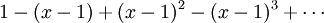# Taylor(Maclaurin) Series – 테일러 급수

In mathematics, the Taylor series is a representation of a function as an infinite sum of terms calculated
from the values of its derivatives at a single point. It may be regarded as the limit of the Taylor polynomials.
Taylor series are named in honour of English mathematician Brook Taylor.
If the series uses the derivatives at zero, the series is also called a Maclaurin series,
named after Scottish mathematician Colin Maclaurin.

The Taylor series of a real or complex function f(x) that is infinitely differentiable in a neighborhood of a real
or complex number a, is the power serieswhich in a more compact form can be written aswhere n! is the factorial of n and f (n)(a) denotes the nth derivative of f at the point a;
the zeroth derivative of f is defined to be f itself and (x − a)^0 and 0! are both defined to be 1.

Often f(x) is equal to its Taylor series evaluated at x for all x sufficiently close to a.
This is the main reason why Taylor series are important.

In the particular case where a = 0, the series is also called a Maclaurin series.

Examples

The Maclaurin series for any polynomial is the polynomial itself.

The Maclaurin series for (1 − x)^(− 1) is the geometric seriesso the Taylor series for x^(− 1) at a = 1 isBy integrating the above Maclaurin series we find the Maclaurin series for -ln(1-x),
where ln denotes the natural logarithm:The Maclaurin series for the exponential function e^x at a = 0 isThe above expansion holds because the derivative of e^x is also e^x and e^0 equals 1.
This leaves the terms (x − 0)^n in the numerator and n! in the denominator for each term in the infinite sum.(As the degree of the Taylor polynomial rises, it approaches the correct function.
This image shows sinx and Taylor approximations, polynomials of degree 1, 3, 5, 7, 9, 11 and 13.)